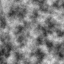tumult
A Javascript noise libraryLast updated a year ago by scottyfillups .
MIT · Repository · Bugs · Original npm · Tarball · package.json
`\$ cnpm install tumult `
SYNC missed versions from official npm registry.

# tumultYet another Javascript noise library. Demonstrations here. Currently supports Perlin noise for any arbitrary dimension and Simplex[1-2]. Eventually might support:

• Simplex[3-4]

## Installation

``````npm install tumult --save
``````

The built files are also available on `unpkg`:

``````<script src="https://unpkg.com/tumult/dist/tumult.min.js"></script>
``````

## Usage

``````const tumult = require('tumult')

const simplex2 = new tumult.Simplex2('some_seed')

for (let x = 0; x < 10; x++) {
for (let y = 0; y < 10; y++) {
console.log(simplex2.gen(x / 64, y / 64))
}
}
``````

## API

### tumult

Object that stores noise constructors. Below is the full list of constructors:

• tumult.Simplex1
• tumult.Simplex2
• tumult.Perlin1
• tumult.Perlin2
• tumult.Perlin3
• tumult.Perlin4
• tumult.PerlinN

Every constructor has the following signature:

#### tumult.NoiseConstructor([seed])

Returns a `noise` object.

#### seed

Type: `String | Number`

Seed to use for shuffling the permutation look-up table. If no value is passed, `Math.random()` will be used as a seed.

### noise

Noise object returned from invoke a noise constructor; all noise objects have the same API:

#### noise.seed([seed])

Re-seeds the permutation look-up table. If a number is passed, it will be converted to a string which will seed the generator. If no string is passed, `.seed()` defaults to using `Math.random()`

#### noise.gen(x, y, z...)

Generates a noise value given the appropriate dimensions (eg. a simplex2 generator should take two arguments, a perlin3 generator should take three arguments, etc.)

#### noise.octavate(octaves, x, y, z...)

Applies fractal Brownian motion, summing iterations of the noise (# of iterations = `octaves`). With each successive iteration, the frequency is doubled and the weight is halved.

Note that the generator created by `tumult.PerlinN` is variadic, meaning you can get Nth dimensional perlin noise by passing N arguments. Note that the gradient lookup table for `perlinN` isn't optimised, so calling `perlinN(x, y)` will likely produce less "attractive" noise than `perlin2(x, y)`.

For quickly displaying heightmaps, I highly recommend using terrapaint.

noise.transform(fn)

Deprecated

``````const tumult = require('tumult')

const simplex2 = new tumult.Simplex2()
const transform = (x, y) => Math.sin(1 / simplex2(x, y))
``````

Takes in a function which will its `this` bound to noiseGenerator object, meaning you can call `gen` and `octavate` using `this.gen`, etc. This function should take in the dimensions as parameters, and return a value. `.transform` will return the new transformed noise function. For example, suppose you want a function which will return `sin(1/noise(x/32,y/32))`, you can do the following:

``````const tumult = require('tumult')

const simplex2 = new tumult.Simplex2('seed')
const noise = simplex2.transform(function (x, y) {
return Math.sin(1 / this.gen(x/32, y/32))
})

for (let i = 0; i < 100; i++) {
for (let j = 0; j < 100; j++) {
console.log(noise(i, j))
}
}
``````

TL;DR, `noise.transform` is essentially a helper function that lets you wrap the noise function with your own function.

## Note on testing

Currently the tests only verify trivial test requirements (eg. presence of methods, checking if output is within expected [-1, 1] bound); a better way to test this library would be to utilize OpenCV to verify the noise produced is correct, outlined here: https://stackoverflow.com/questions/32023240/how-to-write-unit-tests-for-a-perlin-noise-library

Unfortunately I'm lacking the bandwidth to implement this, but pull requests are welcome!

## Acknowledgements

Perlin noise was invented in 1985 by Ken Perlin.

## Current Tags

• 3.0.14                                ...           latest (a year ago)

## 21 Versions

• 3.0.14                                ...           a year ago
• 3.0.13                                ...           a year ago
• 3.0.12                                ...           a year ago
• 3.0.11                                ...           2 years ago
• 3.0.10                                ...           2 years ago
• 3.0.9                                ...           2 years ago
• 1.0.6                                ...           2 years ago
• 1.0.5                                ...           2 years ago
• 3.0.7                                ...           2 years ago
• 1.0.4                                ...           2 years ago
• 1.0.3                                ...           2 years ago
• 1.0.2                                ...           2 years ago
• 1.0.1                                ...           2 years ago
• 3.0.5                                ...           2 years ago
• 3.0.4                                ...           2 years ago
• 3.0.3                                ...           3 years ago
• 3.0.2                                ...           3 years ago
• 3.0.1                                ...           3 years ago
• 3.0.0                                ...           3 years ago
• 2.0.0                                ...           3 years ago
• 1.0.0                                ...           3 years ago